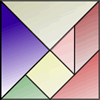Search by Topic

Filter by: Content type:
Age range:
Challenge level:

There are 45 NRICH Mathematical resources connected to Compound transformations, you may find related items under Transformations and constructions.

Broad Topics > Transformations and constructions > Compound transformations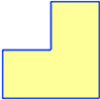Twice as Big?

Age 7 to 11 Challenge Level:

Investigate how the four L-shapes fit together to make an enlarged L-shape. You could explore this idea with other shapes too.Who Is the Fairest of Them All ?

Age 11 to 14 Challenge Level:

Explore the effect of combining enlargements....on the Wall

Age 11 to 14 Challenge Level:

Explore the effect of reflecting in two intersecting mirror lines.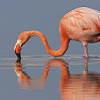Mirror, Mirror...

Age 11 to 14 Challenge Level:

Explore the effect of reflecting in two parallel mirror lines.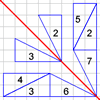Transformation Game

Age 11 to 14 Challenge Level:

Why not challenge a friend to play this transformation game?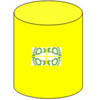Paint Rollers for Frieze Patterns.

Age 11 to 16

Proofs that there are only seven frieze patterns involve complicated group theory. The symmetries of a cylinder provide an easier approach.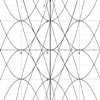Age 14 to 18 Challenge Level:

This task develops knowledge of transformation of graphs. By framing and asking questions a member of the team has to find out which mathematical function they have chosen.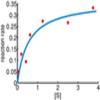Curve Fitter 2

Age 16 to 18 Challenge Level:

Can you construct a cubic equation with a certain distance between its turning points?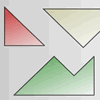Square Tangram

Age 7 to 11 Challenge Level:

This was a problem for our birthday website. Can you use four of these pieces to form a square? How about making a square with all five pieces?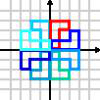Grouping Transformations

Age 11 to 18

An introduction to groups using transformations, following on from the October 2006 Stage 3 problems.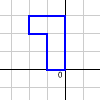Simplifying Transformations

Age 11 to 14 Challenge Level:

How many different transformations can you find made up from combinations of R, S and their inverses? Can you be sure that you have found them all?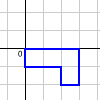Combining Transformations

Age 11 to 14 Challenge Level:

Does changing the order of transformations always/sometimes/never produce the same transformation?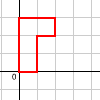Decoding Transformations

Age 11 to 14 Challenge Level:

See the effects of some combined transformations on a shape. Can you describe what the individual transformations do?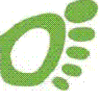Footprints

Age 16 to 18 Challenge Level:

Make a footprint pattern using only reflections.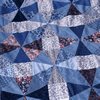Mathematical Patchwork

Age 7 to 14

Jenny Murray describes the mathematical processes behind making patchwork in this article for students.Parallel Parking

Age 14 to 16

Scientist Bryan Rickett has a vision of the future - and it is one in which self-parking cars prowl the tarmac plains, hunting down suitable parking spots and manoeuvring elegantly into them.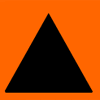Sorting Symmetries

Age 7 to 11 Challenge Level:

Find out how we can describe the "symmetries" of this triangle and investigate some combinations of rotating and flipping it.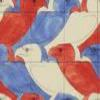Maurits Cornelius Escher

Age 7 to 14

Have you ever noticed how mathematical ideas are often used in patterns that we see all around us? This article describes the life of Escher who was a passionate believer that maths and art can be. . . .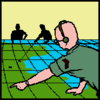Going Places with Mathematicians

Age 7 to 14

This article looks at the importance in mathematics of representing places and spaces mathematics. Many famous mathematicians have spent time working on problems that involve moving and mapping. . . .Squares, Squares and More Squares

Age 11 to 14 Challenge Level:

Can you dissect a square into: 4, 7, 10, 13... other squares? 6, 9, 12, 15... other squares? 8, 11, 14... other squares?Matching Frieze Patterns

Age 11 to 14 Challenge Level:

Sort the frieze patterns into seven pairs according to the way in which the motif is repeated.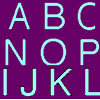Transforming the Letters

Age 7 to 11 Challenge Level:

What happens to these capital letters when they are rotated through one half turn, or flipped sideways and from top to bottom?Bow Tie

Age 11 to 14 Challenge Level:

Show how this pentagonal tile can be used to tile the plane and describe the transformations which map this pentagon to its images in the tiling.Reflect Again

Age 16 to 18 Challenge Level:

Follow hints to investigate the matrix which gives a reflection of the plane in the line y=tanx. Show that the combination of two reflections in intersecting lines is a rotation.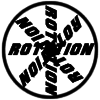Rots and Refs

Age 16 to 18 Challenge Level:

Follow hints using a little coordinate geometry, plane geometry and trig to see how matrices are used to work on transformations of the plane.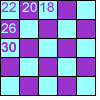Transformations Tables

Age 7 to 11 Challenge Level:

These grids are filled according to some rules - can you complete them?Trees and Friezes

Age 11 to 14 Challenge Level:

This problem is based on the idea of building patterns using transformations.Friezes Using Logo

Age 11 to 14 Challenge Level:

Experimenting with variables and friezes.The Use of Mathematics in Computer Games

Age 16 to 18

An account of how mathematics is used in computer games including geometry, vectors, transformations, 3D graphics, graph theory and simulations.The Frieze Tree

Age 11 to 16

Patterns that repeat in a line are strangely interesting. How many types are there and how do you tell one type from another?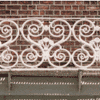Frieze Patterns in Cast Iron

Age 11 to 16

A gallery of beautiful photos of cast ironwork friezes in Australia with a mathematical discussion of the classification of frieze patterns.Making Rectangles, Making Squares

Age 11 to 14 Challenge Level:

How many differently shaped rectangles can you build using these equilateral and isosceles triangles? Can you make a square?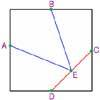Midpoint Triangle

Age 7 to 11 Challenge Level:

Can you cut up a square in the way shown and make the pieces into a triangle?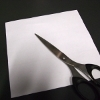Square to L

Age 7 to 11 Challenge Level:

Find a way to cut a 4 by 4 square into only two pieces, then rejoin the two pieces to make an L shape 6 units high.Flight of the Flibbins

Age 11 to 14 Challenge Level:

Blue Flibbins are so jealous of their red partners that they will not leave them on their own with any other bue Flibbin. What is the quickest way of getting the five pairs of Flibbins safely to. . . .Triangular Tantaliser

Age 11 to 14 Challenge Level:

Draw all the possible distinct triangles on a 4 x 4 dotty grid. Convince me that you have all possible triangles.Counting Triangles

Age 11 to 14 Challenge Level:

Triangles are formed by joining the vertices of a skeletal cube. How many different types of triangle are there? How many triangles altogether?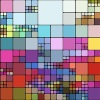Squaring the Rectangle

Age 14 to 18 Challenge Level:

Can you find a way to turn a rectangle into a square?Screwed-up

Age 11 to 14 Challenge Level:

A cylindrical helix is just a spiral on a cylinder, like an ordinary spring or the thread on a bolt. If I turn a left-handed helix over (top to bottom) does it become a right handed helix?2001 Spatial Oddity

Age 11 to 14 Challenge Level:

With one cut a piece of card 16 cm by 9 cm can be made into two pieces which can be rearranged to form a square 12 cm by 12 cm. Explain how this can be done.Chess

Age 11 to 14 Challenge Level:

What would be the smallest number of moves needed to move a Knight from a chess set from one corner to the opposite corner of a 99 by 99 square board?Sine Problem

Age 16 to 18 Challenge Level:

In this 'mesh' of sine graphs, one of the graphs is the graph of the sine function. Find the equations of the other graphs to reproduce the pattern.Cut and Make

Age 7 to 11 Challenge Level:

Cut a square of paper into three pieces as shown. Now,can you use the 3 pieces to make a large triangle, a parallelogram and the square again?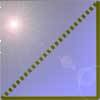Cutting Corners

Age 7 to 11 Challenge Level:

Can you make the most extraordinary, the most amazing, the most unusual patterns/designs from these triangles which are made in a special way?